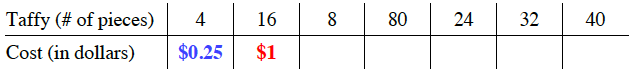### Home > MC2 > Chapter 7 > Lesson 7.2.3 > Problem7-87

7-87.

Four pieces of taffy cost $25$ cents. Complete the table below.

 Taffy (# of pieces) Cost (in dollars) $4$ $16$ $8$ $80$ $24$ $32$ $40$

What can you multiply $4$ by to get $16$ pieces of taffy?

$0.25 \left(4\right) = 1$ dollar for $16$ pieces of taffyNow use this same method to fill in the rest of the table.